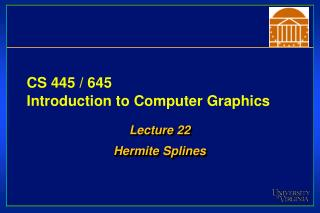DownloadDownload PresentationCS 445 / 645 Introduction to Computer Graphics

# CS 445 / 645 Introduction to Computer Graphics

Download Presentation## CS 445 / 645 Introduction to Computer Graphics

- - - - - - - - - - - - - - - - - - - - - - - - - - - E N D - - - - - - - - - - - - - - - - - - - - - - - - - - -
##### Presentation Transcript

1. CS 445 / 645Introduction to Computer Graphics Lecture 22 Hermite Splines

2. Splines – Old School Duck Spline

3. Representations of Curves • Use a sequence of points… • Piecewise linear - does not accurately model a smooth line • Tedious to create list of points • Expensive to manipulate curve because all points must be repositioned • Instead, model curve as piecewise-polynomial • x = x(t), y = y(t), z = z(t) • where x(), y(), z() are polynomials

4. Specifying Curves(hyperlink) • Control Points • A set of points that influence the curve’s shape • Knots • Control points that lie on the curve • Interpolating Splines • Curves that pass through the control points (knots) • Approximating Splines • Control points merely influence shape

5. Parametric Curves • Very flexible representation • They are not required to be functions • They can be multivalued with respect to any dimension

6. Cubic Polynomials • x(t) = axt3 + bxt2 + cxt + dx • Similarly for y(t) and z(t) • Let t: (0 <= t <= 1) • Let T = [t3 t2 t 1] • Coefficient Matrix C • Curve: Q(t) = T*C

7. Another nice feature of curves • Derivatives • Very useful for lighting equations • Useful for automatic feature detection

8. Parametric Curves • How do we find the tangent to a curve? • If f(x) = • tangent at (x=3) is • Derivative of Q(t) is the tangent vector at t:

9. Piecewise Curve Segments • One curve constructed by connecting many smaller segments end-to-end • Must have rules for how the segments are joined • Continuity describes the joint • Parametric continuity • Geometric continuity

10. Parametric Continuity • C1 is tangent continuity (velocity) • C2 is 2nd derivative continuity (acceleration) • Matching direction and magnitude of dn / dtn • Cn continous

11. Geometric Continuity • If positions match • G0 geometric continuity • If direction (but not necessarily magnitude) of tangent matches • G1 geometric continuity • The tangent value at the end of one curve is proportional to the tangent value of the beginning of the next curve

12. Parametric Cubic Curves • In order to assure C2 continuity, curves must be of at least degree 3 • Here is the parametric definition of a cubic (degree 3) spline in two dimensions • How do we extend it to three dimensions?

13. Parametric Cubic Splines • Can represent this as a matrix too

14. Coefficients • So how do we select the coefficients? • [ax bx cx dx] and [ay by cy dy] must satisfy the constraints defined by the knots and the continuity conditions

15. Parametric Curves • Difficult to conceptualize curve as x(t) = axt3 + bxt2 + cxt + dx(artists don’t think in terms of coefficients of cubics) • Instead, define curve as weighted combination of 4 well-defined cubic polynomials(wait a second! Artists don’t think this way either!) • Each curve type defines different cubic polynomials and weighting schemes

16. Parametric Curves • Hermite – two endpoints and two endpoint tangent vectors • Bezier - two endpoints and two other points that define the endpoint tangent vectors • Splines – four control points • C1 and C2 continuity at the join points • Come close to their control points, but not guaranteed to touch them • Examples of Splines

17. Hermite Cubic Splines • An example of knot and continuity constraints

18. Hermite Cubic Splines • One cubic curve for each dimension • A curve constrained to x/y-plane has two curves:

19. Hermite Cubic Splines • A 2-D Hermite Cubic Spline is defined by eight parameters: a, b, c, d, e, f, g, h • How do we convert the intuitive endpoint constraints into these (relatively) unintuitive eight parameters? • We know: • (x, y) position at t = 0, p1 • (x, y) position at t = 1, p2 • (x, y) derivative at t = 0, dp/dt • (x, y) derivative at t = 1, dp/dt

20. Hermite Cubic Spline • We know: • (x, y) position at t = 0, p1

21. Hermite Cubic Spline • We know: • (x, y) position at t = 1, p2

22. Hermite Cubic Splines • So far we have four equations, but we have eight unknowns • Use the derivatives

23. Hermite Cubic Spline • We know: • (x, y) derivative at t = 0, dp/dt

24. Hermite Cubic Spline • We know: • (x, y) derivative at t = 1, dp/dt

25. Hermite Specification • Matrix equation for Hermite Curve t3 t2 t1 t0 t = 0 p1 t = 1 p2 t = 0 r p1 r p2 t = 1

26. Solve Hermite Matrix

27. Spline and Geometry Matrices MHermite GHermite

28. Resulting Hermite Spline Equation

29. Demonstration • Hermite

30. Sample Hermite Curves

31. Blending Functions • By multiplying first two matrices in lower-left equation, you have four functions of ‘t’ that blend the four control parameters • These are blendingfunctions

32. Hermite Blending Functions • If you plot the blending functions on the parameter ‘t’

33. Hermite Blending Functions • Remember, eachblending functionreflects influenceof P1, P2, DP1, DP2on spline’s shape